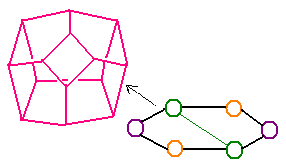Recall that the faces of an associahedron are labelled by a polygon with one chord. In real dimension $3$ we have hexagons, for which there are two chord types.The square faces of the associahedron are marked by a hexagon of the kind shown. Since Loday's trefoil has crossings on these squares, each knot crossing corresponds to a pair of vertices on the hexagon. In M theory, we sometimes label these vertices by $(X, -X)$, or perhaps $\pm \sigma_{X}$, and so on. Note that a trefoil describes the quandle (or rack) rules for the Pauli operators.